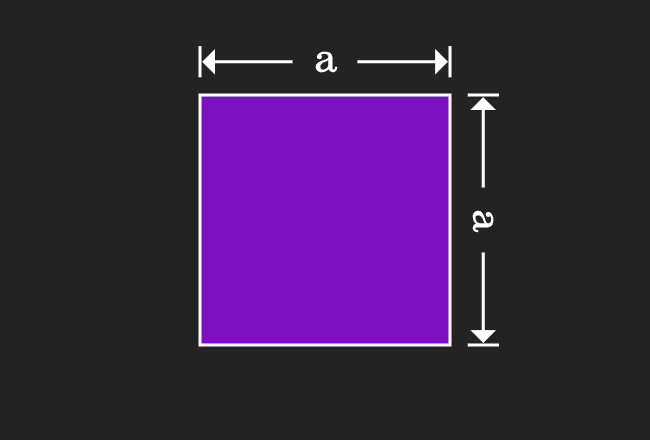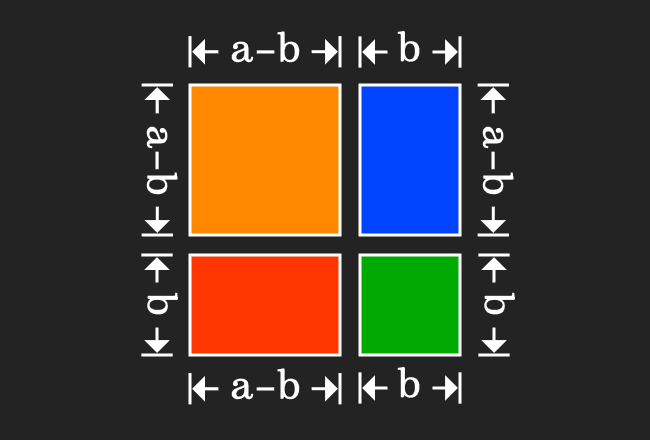# Proof of $(a-b)^2$ formula in Geometrical Method

The expansion of a minus b whole squared algebraic identity can be derived in algebraic form by the geometrical approach. The concept of areas of geometrical shapes such as squares and rectangles are used for proving the a minus b whole square formula in algebraic form.

### Find the Area of a squareTake a square and assume the length of each side of this square is represented by $a$.

We have to calculate the area of this geometric shape mathematically. According to the mathematics, the area of a square is equal to the square of the length of the side.

In this case, it is taken that the length of the each side is equal to $a$. Therefore, the area of this square is equal to $a^2$, which is very important to remember for deriving the $a-b$ whole square algebraic identity.

### Basic steps to split the square

For proving the $a$ minus $b$ whole squared formula geometrically, we have to split the square as four different geometrical figures.1. Draw a straight line vertically across the pair of opposite sides for dividing the square as two rectangles. If the length of one rectangle is denoted by $b$, then the length of remaining rectangle will be $a-b$.
2. Divide the both rectangles horizontally by drawing a straight line such that the width of two shapes are equal to $b$, then the widths of remaining two shapes are equal to $a-b$. It is useful for creating a square, whose area is equal to $a-b$ whole square.

Geometrically, we have successfully divided a square as two small squares and two rectangles.

### Evaluate the Areas of Geometric shapes

Now, calculate the area of each geometrical shape mathematically.1. The length of each side of one square is $a-b$, then its area is equal to $(a-b)^2$.
2. The length and width of one rectangle are $b$ and $a-b$ respectively, then its area is equal to $b \times (a-b)$, which can be written as $b(a-b)$ simply.
3. The length and width of second rectangle are $a-b$ and $b$ respectively, then its area is equal to $(a-b) \times b$, which is simply written as $(a-b)b$ in mathematics.
4. The length of the remaining square is $b$. Therefore, its area is equal to $b^2$.

Thus, the areas of all geometrical shapes are calculated and expressed in algebraic form.

### Equality of the Areas of the Geometric shapes

It is time to prove the expansion of the $a$ minus $b$ whole squared formula geometrically.Geometrically, a square is divided as four different geometrical shapes. So, the area of the square is equal to the sum of the areas of the four geometrical shapes. It can be written in mathematical form as an equation.

$a^2$ $\,=\,$ $(a-b)^2$ $+$ $b(a-b)$ $+$ $(a-b)b$ $+$ $b^2$

In this derivation, we have to find the expansion of $(a-b)^2$ identity. So, shift all the terms to other side of the equation for finding the equivalent value of the $a-b$ whole squared.

$\implies$ $a^2$ $-$ $b(a-b)$ $-$ $(a-b)b$ $-$ $b^2$ $\,=\,$ $(a-b)^2$

$\implies$ $(a-b)^2$ $\,=\,$ $a^2$ $-$ $b(a-b)$ $-$ $(a-b)b$ $-$ $b^2$

In the right hand side of the equation, the second and third terms $b(a-b)$ and $(a-b)b$ are equal mathematically as per commutative property of multiplication.

$\implies$ $(a-b)^2$ $\,=\,$ $a^2$ $-$ $b(a-b)$ $-$ $b(a-b)$ $-$ $b^2$

$\implies$ $(a-b)^2$ $\,=\,$ $a^2-2b(a-b)-b^2$

$\implies$ $(a-b)^2$ $\,=\,$ $a^2-2ba+2b^2-b^2$

$\,\,\, \therefore \,\,\,\,\,\,$ $(a-b)^2$ $\,=\,$ $a^2-2ab+b^2$

It is also written as $(a-b)^2$ $\,=\,$ $a^2+b^2-2ab$

It is read as the $a$ minus $b$ whole squared is equal to $a$ squared plus $b$ squared minus $2$ times product of $a$ and $b$. Thus, the $a-b$ whole square algebraic identity is proved in algebraic form geometrically.

Latest Math Topics
Jun 26, 2023
Jun 23, 2023

Latest Math Problems
Jul 01, 2023
Jun 25, 2023
###### Math Questions

The math problems with solutions to learn how to solve a problem.

Learn solutions

Practice now

###### Math Videos

The math videos tutorials with visual graphics to learn every concept.

Watch now

###### Subscribe us

Get the latest math updates from the Math Doubts by subscribing us.